# Graph problems Partition min cut Clustering bioinformatics Image

• Slides: 25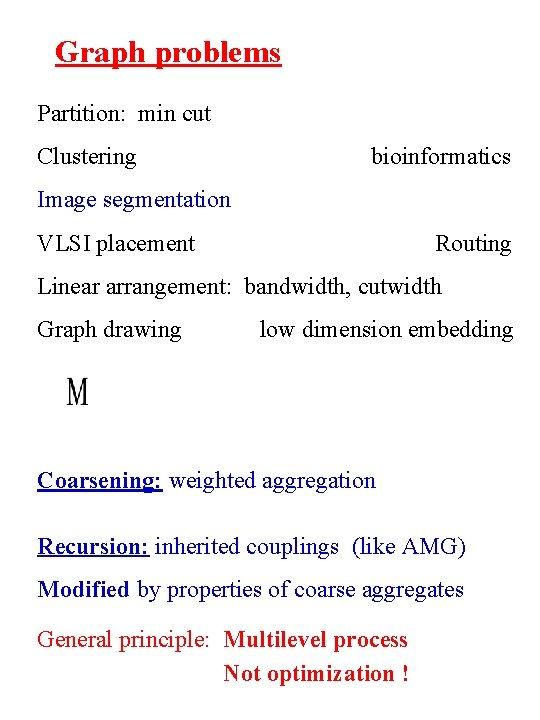Graph problems Partition: min cut Clustering bioinformatics Image segmentation VLSI placement Routing Linear arrangement: bandwidth, cutwidth Graph drawing low dimension embedding Coarsening: weighted aggregation Recursion: inherited couplings (like AMG) Modified by properties of coarse aggregates General principle: Multilevel process Not optimization !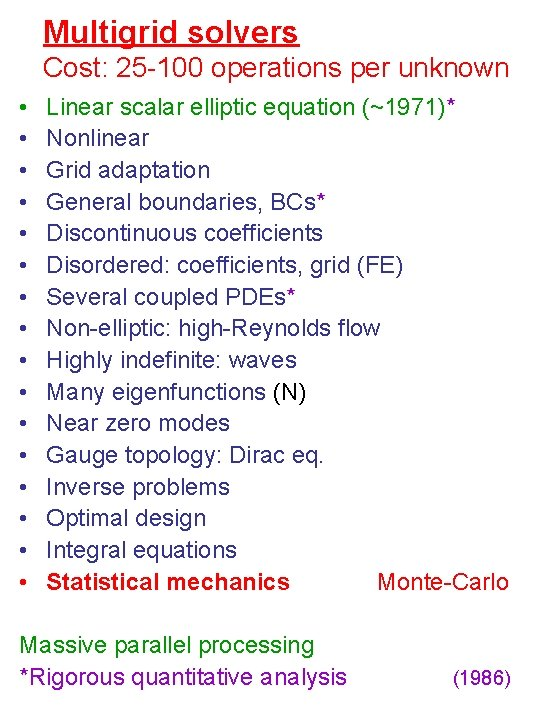Multigrid solvers Cost: 25 -100 operations per unknown • • • • Linear scalar elliptic equation (~1971)* Nonlinear Grid adaptation General boundaries, BCs* Discontinuous coefficients Disordered: coefficients, grid (FE) Several coupled PDEs* Non-elliptic: high-Reynolds flow Highly indefinite: waves Many eigenfunctions (N) Near zero modes Gauge topology: Dirac eq. Inverse problems Optimal design Integral equations Statistical mechanics Monte-Carlo Massive parallel processing *Rigorous quantitative analysis (1986)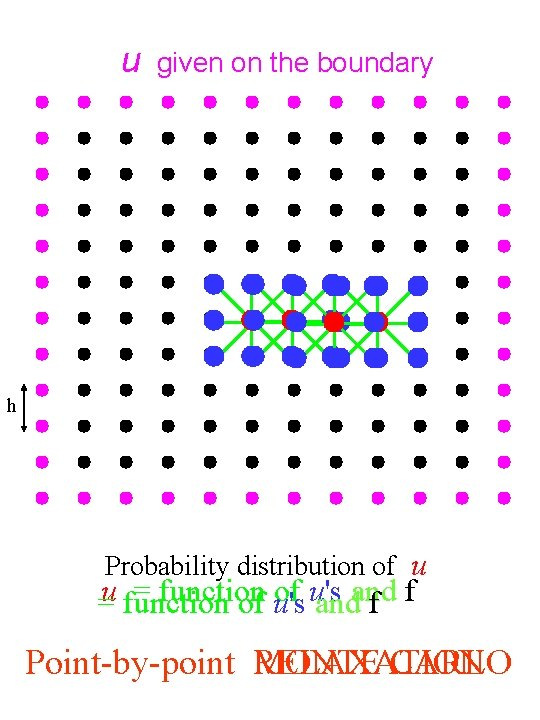u given on the boundary h Probability distribution of u = function of u's =u function of u's andand f f Point-by-point RELAXATION MONTE CARLO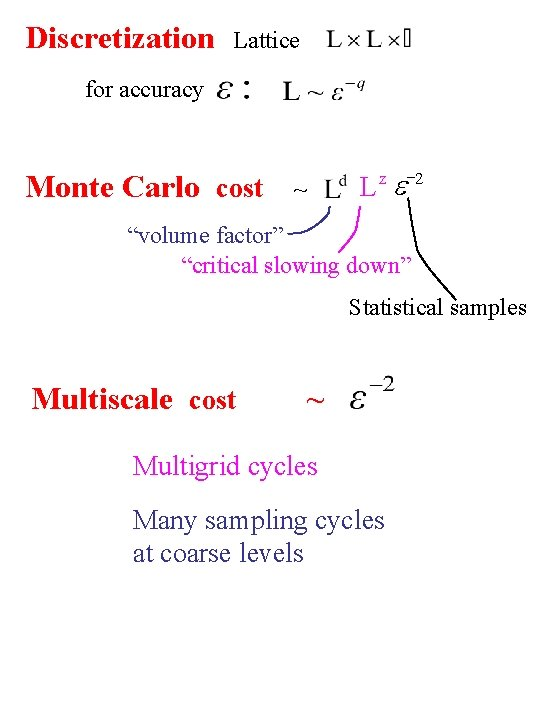Discretization Lattice for accuracy Monte Carlo cost -2 e L z ~ “volume factor” “critical slowing down” Statistical samples Multiscale cost ~ Multigrid cycles Many sampling cycles at coarse levels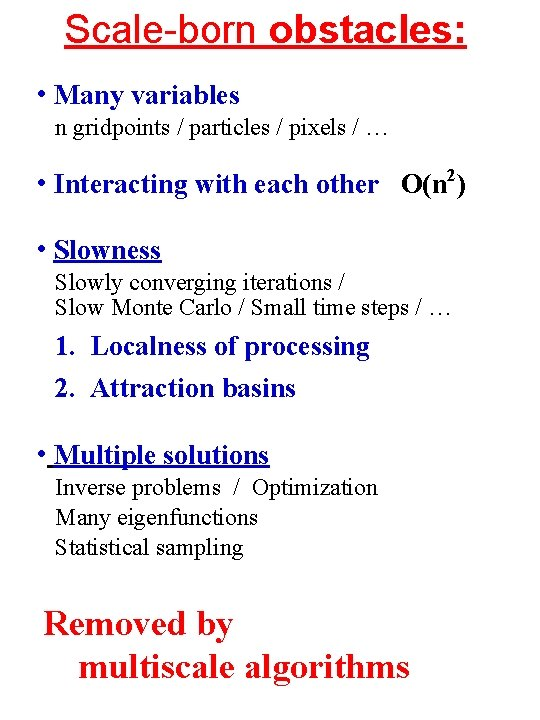Scale-born obstacles: • Many variables n gridpoints / particles / pixels / … • Interacting with each other O(n 2) • Slowness Slowly converging iterations / Slow Monte Carlo / Small time steps / … 1. Localness of processing 2. Attraction basins • Multiple solutions Inverse problems / Optimization Many eigenfunctions Statistical sampling Removed by multiscale algorithms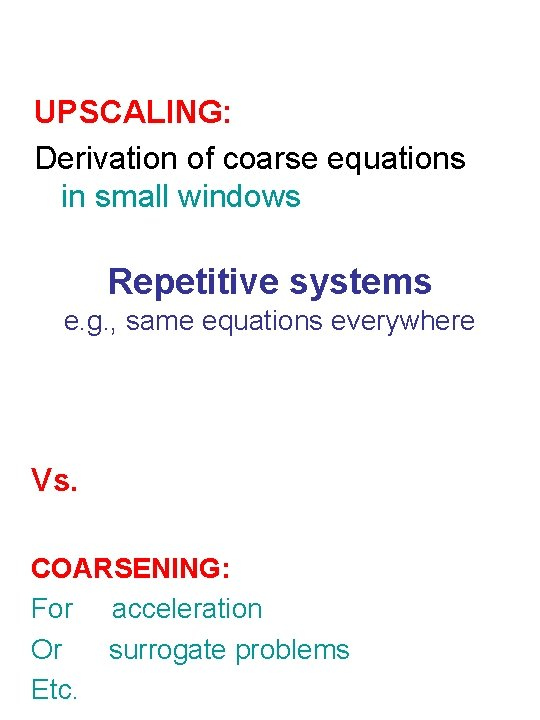UPSCALING: Derivation of coarse equations in small windows Repetitive systems e. g. , same equations everywhere Vs. COARSENING: For acceleration Or surrogate problems Etc.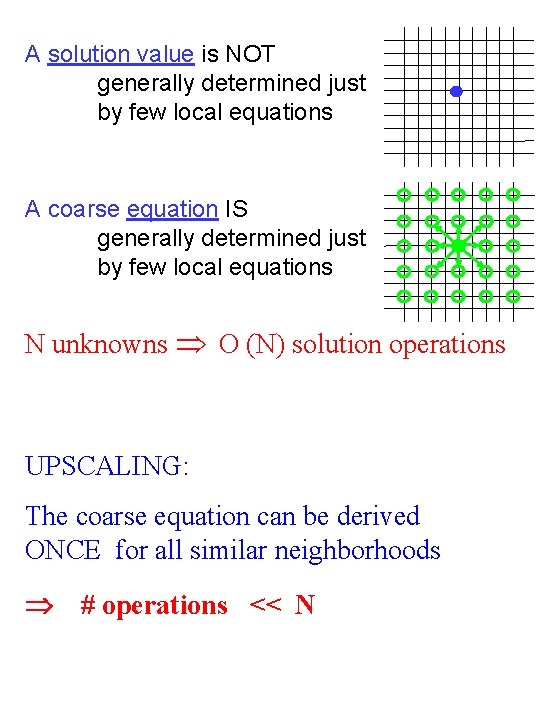A solution value is NOT generally determined just by few local equations A coarse equation IS generally determined just by few local equations N unknowns O (N) solution operations UPSCALING: The coarse equation can be derived ONCE for all similar neighborhoods # operations << N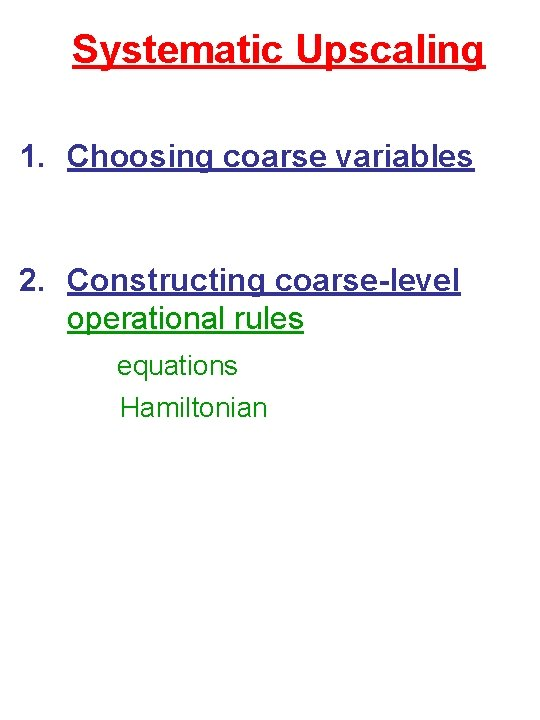Systematic Upscaling 1. Choosing coarse variables 2. Constructing coarse-level operational rules equations Hamiltonian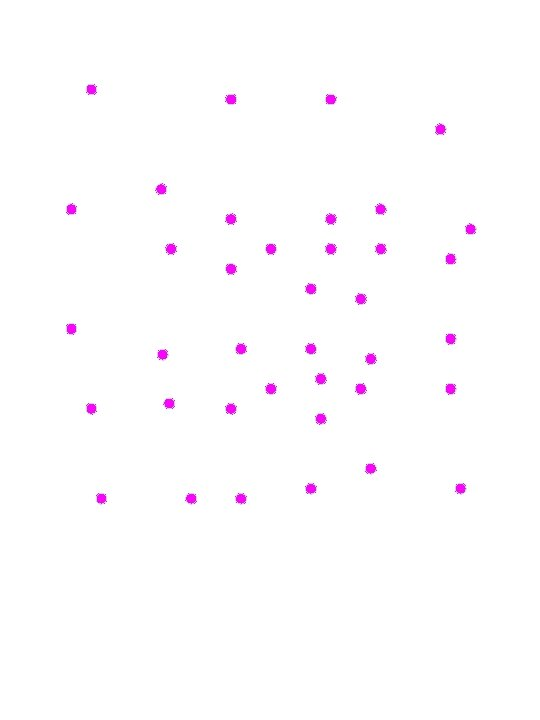Macromolecule ~ 10 -15 second stepsALGEBRAIC MULTIGRID (AMG) 1982 Ax = b Coarse variables - a subset Criterion: Fast convergence of “compatible relaxation” Relax Ax = 0 Keeping coarse variables = 0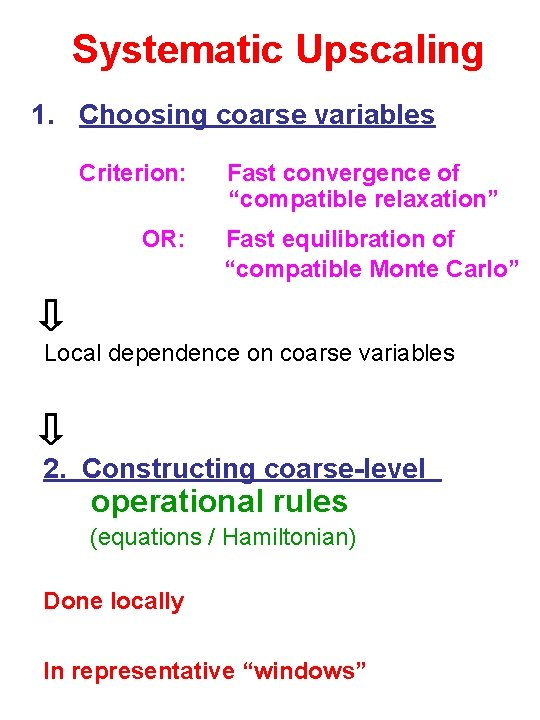Systematic Upscaling 1. Choosing coarse variables Criterion: OR: Fast convergence of “compatible relaxation” Fast equilibration of “compatible Monte Carlo” Local dependence on coarse variables 2. Constructing coarse-level operational rules (equations / Hamiltonian) Done locally In representative “windows”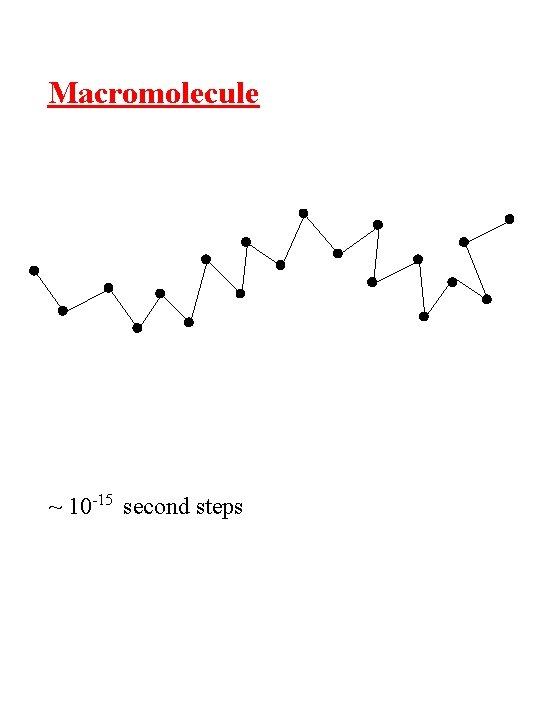Macromolecule ~ 10 -15 second stepsMacromolecule Two orders of magnitude faster simulationMacromolecule t Dihedral potential G 1 -p G 2 T p t 0 + Lennard-Jones + Electrostatic ~104 Monte Carlo passes for one T Gi transitionFluids £ £ Total mass Total momentum Total dipole moment average location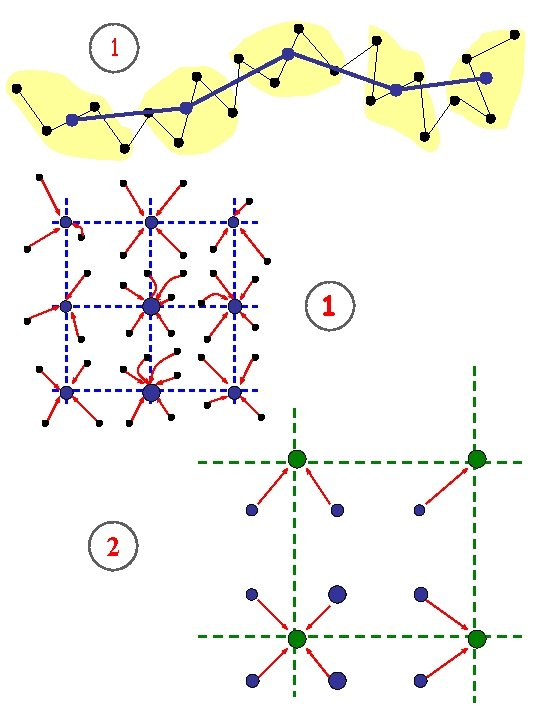1 1 2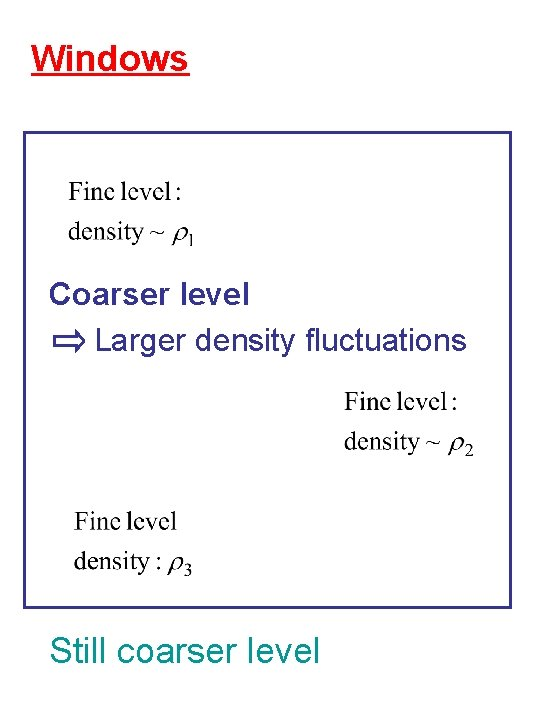Windows Coarser level Larger density fluctuations Still coarser level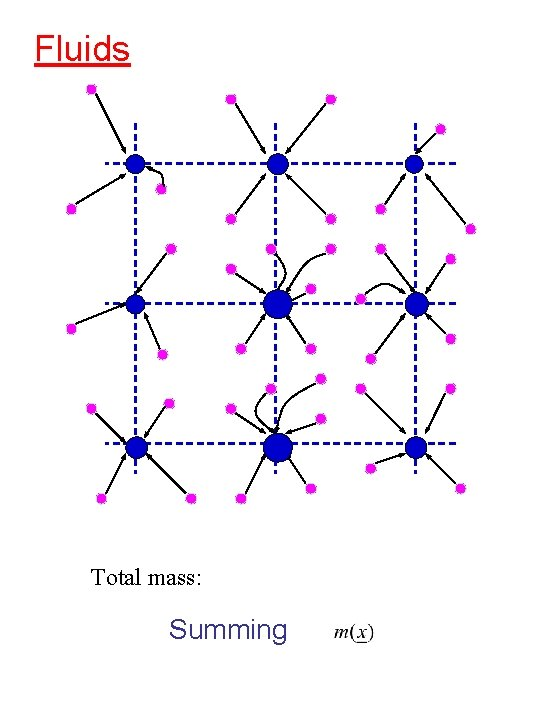Fluids Total mass: SummingLower Temperature T Summing also Still lower T: More precise crystal direction and periods determined at coarser spatial levels Heisenberg uncertainty principle: Better orientational resolution at larger spatial scalesOptimization by Multiscale annealing Identifying increasingly larger-scale degrees of freedom at progressively lower temperatures Handling multiscale attraction basins E(r) r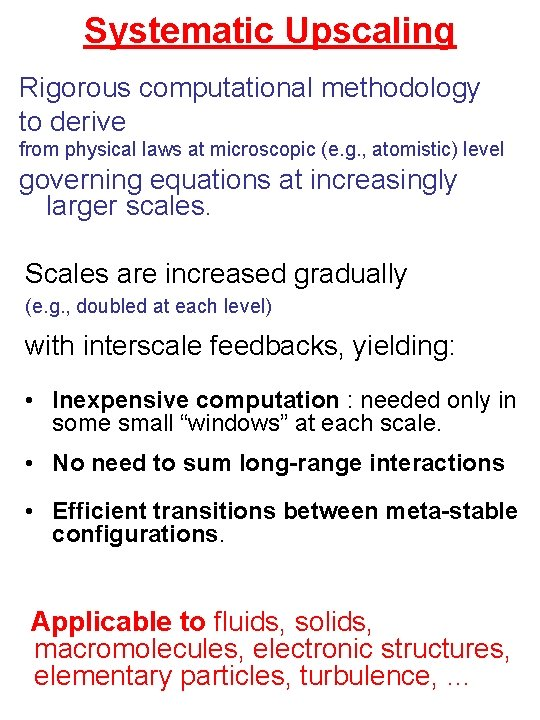Systematic Upscaling Rigorous computational methodology to derive from physical laws at microscopic (e. g. , atomistic) level governing equations at increasingly larger scales. Scales are increased gradually (e. g. , doubled at each level) with interscale feedbacks, yielding: • Inexpensive computation : needed only in some small “windows” at each scale. • No need to sum long-range interactions • Efficient transitions between meta-stable configurations. Applicable to fluids, solids, macromolecules, electronic structures, elementary particles, turbulence, …Upscaling Projects • QCD (elementary particles): Renormalization multigrid BAMG solver of Dirac eqs. Fast update of , det Ron Livne, Livshits Rozantsev • (3 n +1) dimensional Schrödinger eq. Filinov Real-time Feynmann path integrals Zlochin multiscale electronic-density functional • DFT electronic structures Livne, Livshits molecular dynamics • Molecular dynamics: Fluids Polymers, proteins Micromechanical structures Ilyin, Suwain, Makedonska Bai, Klug ? ? ? defects, dislocations, grains • Navier Stokes Turbulence Mc. Williams Dinar, DiskinTHANK YOU